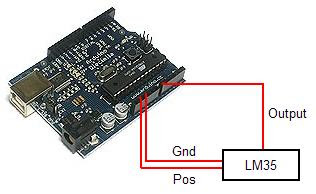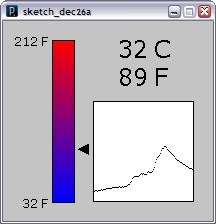## 24 Ekim 2012 Çarşamba

### Arduino , LM35 Sensor and Processing languageMicrocontroller in conjunction with the Processing open source programming environment to monitor temperature.

The project contains 3 parts:
1. The Arduino board with sensor circuit.
2. The Arduino program.
3. The Processing program.

The Arduino Board with Sensor Circuit
The Arduino circuit board is connected to the LM35 Celsius Temperature sensor. Here is a picture of the project circuit with illustrated wires connected to the temperature sensor.

We used the on board power source (5v and Gnd) to power the LM35 and analog pin 0 (zero) to read the analog output from the sensor. Here's a picture of the circuit wired on a breadboard.The LM35 temperature sensor's pin-out and package information is as follows:The Arduino Program

The open-source Arduino environment allows us to write code and load it onto the Arduino board's memory. The development environment is written in Java and based on Processing, avr-gcc, and other open source software.

The Arduino code loops every second to read output from the LM35, converting the analog output into Celsius and sending the data to the computer via a serial communication connection (USB).

Here's the code used to run the Arduino board:

```//declare variables
float tempC;
int tempPin = 0;

void setup()
{
Serial.begin(9600); //opens serial port, sets data rate to 9600 bps
}

void loop()
{
tempC = (5.0 * tempC * 100.0)/1024.0;  //convert the analog data to temperature
Serial.print((byte)tempC);             //send the data to the computer
delay(1000);                           //wait one second before sending new data
}```
The Processing Program
The software client portion of this project runs on a PC and is written in Processing. Processing is a language and development environment similar to Arduino and designed for creating visual effects programs. We used Processing to create a small client that can read the serial data from the Arduino board and display the temperature on a slider and in both Celsius and Fahrenheit. We also added a rolling 100 data point graph to display historical temperature data. Here's a screen shot of the Processing application:Here is the code used for the visual portion of the project:
```//import Serial communication library
import processing.serial.*;

//init variables
Serial commPort;
float tempC;
float tempF;
int yDist;
PFont font12;
PFont font24;
float[] tempHistory = new float;

void setup()
{
//setup fonts for use throughout the application

//set the size of the window
size(210, 200);

//init serial communication port
commPort = new Serial(this, "COM10", 9600);

//fill tempHistory with default temps
for(int index = 0; index<100; index++)
tempHistory[index] = 0;
}

void draw()
{
//get the temp from the serial port
while (commPort.available() > 0)
{

//refresh the background to clear old data
background(123);

//draw the temp rectangle
colorMode(RGB, 160);  //use color mode sized for fading
stroke (0);
rect (49,19,22,162);
//fade red and blue within the rectangle
for (int colorIndex = 0; colorIndex <= 160; colorIndex++)
{
stroke(160 - colorIndex, 0, colorIndex);
line(50, colorIndex + 20, 70, colorIndex + 20);
}

//draw graph
stroke(0);
fill(255,255,255);
rect(90,80,100,100);
for (int index = 0; index<100; index++)
{
if(index == 99)
tempHistory[index] = tempC;
else
tempHistory[index] = tempHistory[index + 1];
point(90 + index, 180 - tempHistory[index]);
}

//write reference values
fill(0,0,0);
textFont(font12);
textAlign(RIGHT);
text("212 F", 45, 25);
text("32 F", 45, 187);

//draw triangle pointer
yDist = int(160 - (160 * (tempC * 0.01)));
stroke(0);
triangle(75, yDist + 20, 85, yDist + 15, 85, yDist + 25);

//write the temp in C and F
fill(0,0,0);
textFont(font24);
textAlign(LEFT);
text(str(int(tempC)) + " C", 115, 37);
tempF = ((tempC*9)/5) + 32;
text(str(int(tempF)) + " F", 115, 65);
}
}```